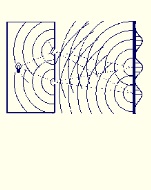Enter the content which will be displayed in sticky bar

# BookPublisher: Les Editions du Nordir
Year: 1993/1995

Absurdities in Modern Physics: A Solution

When I chose to study physics, I thought that science was always rational. Modern physics has certainly failed to fulfill those expectations. For example, I found that the widely accepted Copenhagen interpretation does not allow us to believe in the real existence of matter and that the law of causality is not applied in quantum theory. David Layzer gave one of the most honest descriptions of modern physics when he said that modern physics is merely a computational device for predicting the outcomes of possible measurements. Unfortunately, his statement is true. Physics can be studied from many different points of view. Its aim can be to make numerical predictions of some phenomena or to present a rational way of explaining physical observations. These are two quite different aspects. Let us consider the first aspect predicting events. Using mathematical equations, modern physics can make predictions with extreme reliability. The mathematical formalism used in physics is so powerful that, when it leads to cases that can be calculated, it gives predictions that are compatible with all known experiments. This book does not directly deal with mathematical physics. Therefore, we will avoid using, as much as possible, most of the equations known in modern physics. We do not challenge any of the mathematical equations of modern physics. The usual aspect of physics, as being a computational device for predicting the outcomes of possible measurements, is not considered here at all. In this book, we consider only the second aspect of physics. When we deal with physics, we must ask: Do rules other than the ones imposed by mathematical logic exist? Yes, there are in physics some elements that do not exist in mathematics. Physics deals with concepts such as mass, length, time and energy. These concepts correspond in our mind to images different from the ones represented by mathematical relations. They have a different representation in our mind because they must be submitted to external tests. They have to comply with observational results. There is no equivalence in mathematics. A mathematical demonstration never implies any experimentation. Mathematics simply deals with the calculation of relations between those concepts.

Once available from R. Yergeau, Simard Hall, 165 Waller Street, Ottawa, ON K1J 7N4, CANADA at \$20.